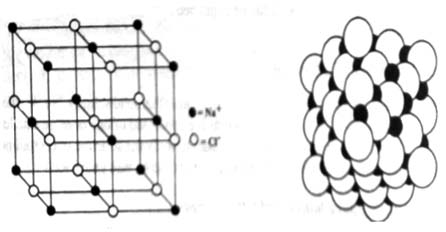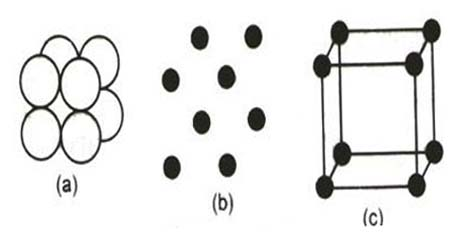Chemistry

# Internal Structure of Crystal: Crystal lattice, Space Lattice and Lattice PointsInternal Structure of a Crystal

We know that solid substances can either be crystalline or amorphous. The crystalline solids are composed of one or more smaller crystals each having a well-ordered structure. In order to study the structure of crystalline solids certain terms commonly used in crystallography should be well understood.

Crystal lattice, space lattice and lattice Points

The definite geometric shape of the crystals results from complete regular arrangements of atoms, molecules or ions in three dimensions. This three dimensional ordered arrangement of one or more smaller units of crystals is conveniently described in terms of a crystal lattice. The lattice is divided into a number of identical blocks or cells that are repeated in all directions to form a particular geometric pattern. Lattices are classified according to their dominant symmetries. These are trigonal, hexagonal, tetrahedral, orthorhombic, monoclinic, and triclinic. However, the concept of crystal lattice can be best understood by considering two-dimensional repeating units as shown in Figure (A).

It can be seen from the figure that a pattern of A’s has been demonstrated which consists of basic repeating units. A lattice point is obtained by selecting the same point (a dot at the crossbar of each A) in each of the same basic unit (in this case each A). Each point in the lattice has exactly the same environment as any similar point. The collection of these points (i.e. the dots) constitutes the pattern of the lattice points (Figure: B). The pattern of points in space is known as the space lattice. Compounds that exhibit a crystal lattice structure include sodium chloride (table salt), calcium chloride, boron nitride etc.Figure 1: A two dimensional representation of a crystal lattice.

(A) A pattern of A’s (A’s may be an atom, molecule or ion).

(B) The pattern of lattice points.

(C) Subdivision of crystal lattice into unit cells and

Let consider the space lattice of NaCl (Figure 2). Each of the points corresponds to the position of the centre of an ion.  indicates Na+ ions and o indicates Cl ions. The points do not represent sodium ions or chloride ions but the positions occupied by their centres. In fact in sodium chloride the tons are of different sizes and are practically touching as shown in Figure 3.Fig 1: The sodium chloride lattice

Fig 2: Actual crystal lattice of sodium chloride: black spheres: Sodium ions and hollows spheres; chlorides ions.